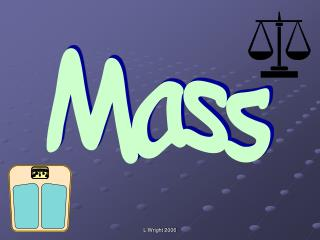DownloadDownload PresentationMass

# Mass

Télécharger la présentation## Mass

- - - - - - - - - - - - - - - - - - - - - - - - - - - E N D - - - - - - - - - - - - - - - - - - - - - - - - - - -
##### Presentation Transcript

1. Mass L Wright 2006

2. Today we will be learning to: • solve measurement problems • use words about mass and ways of measuring weight • measure using non-standard units • compare weights • estimate and measure. L Wright 2006

3. Mental Activity Imagine a spider is on number 4 of a hundred square. Which number is below it? L Wright 2006

4. L Wright 2006

5. Imagine a spider is on number 19 of a hundred square. Which number is below it? Which number is above it? L Wright 2006

6. L Wright 2006

7. Imagine a spider is on number 45 of a hundred square. Which number is below it? Which number is above it? L Wright 2006

8. L Wright 2006

9. Imagine a spider is on number 99 of a hundred square. Which number is above it? L Wright 2006

10. L Wright 2006

11. Imagine a spider is on number 34 of a hundred square. Which number is below it? Which number is above it? L Wright 2006

12. L Wright 2006

13. Main Activity Today we are going to be finding out about mass which is how heavy things are. L Wright 2006

14. I will show you two parcels. Which is the heaviest? How could we find out? What if the parcels were in a different room or place? L Wright 2006

15. You will now be split into six groups. Each group will be given a parcel. How could you find out which is the heaviest parcel? L Wright 2006

16. Each group will be given a balance and some non-standard units. Remember to measure using the same units. L Wright 2006

17. Which unit do you need most of? Why? Which unit is the best or worst for measuring with? Why? L Wright 2006

18. Which parcels were the heaviest? Did you find them all the same? Each parcel weighed 1kg. What does this term mean? Where have you heard the term before? L Wright 2006

19. 1kg lighter heavier Group Work Work in the same groups. Draw a table of classroom objects that are heavier or lighter than a kilogram. L Wright 2006

20. Plenary • Look at the sheet showing a range of different scales. • What step is the scale going up in? • How did you work it out? • What does each division represent? L Wright 2006

21. Remember! • Work out what each division stands for. • Use what you know to work out what you don’t know. Homework Find three things at home which weight more than 1kg and three things which weigh less than 1kg. L Wright 2006